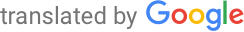Machine-translated page for increased accessibility for English questioners.

# Parallel and distributed systems

1. Graphs and graph algorithms. Formalization of basic graph concepts, representation of graphs. Coherence of a graph, chromaticity, planar graphs. Algorithms (including the complexity and basic idea of proofs of correctness): searching the graph in width and depth, the shortest distances, skeletons, flows in networks.
`MA010, MA015`
2. Mathematical logic. Propositional and predicate logic, syntax, semantics. Derivation systems, formal evidence. Correctness and completeness of derivation systems. Gödel's incompleteness theorems.
`MA007`
3. Finite automata (FA) and logics over words. 1st order logic (FOL) and 2nd order monadic logic (MSOL): syntax and semantics of FOL and MSOL, principles of transferability between FA and MSOL formulas. Automata over infinite words and omega-regular languages.
`IA006`
4. Operational, denotative, and axiomatic semantics of programming languages. CPO, fixed point theorem and its application. Hoare's logic, its correctness and completeness. Temporal logics of linear and branching time and their fragments, semantics of unfinished and parallel programs.
`IA011, IA040 `
5. Model verification (MC) method for finite state systems and linear temporal logic. The principle of translating LTL formulas into automata over infinite words. Basic symbolic and explicit algorithms for MC and their theoretical complexity.
`IA159`
6. Formalisms for modeling infinite state systems (Petri nets, process rewriting systems, automata, process calculations) and process algebras, comparison of their expressive power with respect to bisimulation. Selected decidable problems in the field of verification of these systems.
`IA006, IA023`
7. Specific techniques for verification of software systems, abstract interpretation, methods of abstraction and approximation, reduction by partial arrangement, methods of refinement of abstractions (eg CEGAR - counterexample-controlled refinement of abstractions).
`IA159`
8. Real time systems. Soft and hard systems. Planning in real-time systems: scheduling with periodic tasks, scheduling based on priorities, access to shared resources.
`IA158`
9. Models of distributed systems - basic concepts and principles, synchronous and asynchronous communication. Synchronization. Termination detection. The problem of mutual exclusion and the problem of deadlocks and their solutions. The problem of choosing the leading element - the influence of topology and its knowledge / ignorance on the complexity of solving the problem.
`IV100`
10. Computer networks - basic concepts, principles, architectures. Connected and unconnected networks, OSI model, protocols in the Internet environment. Routing, basic computer network services, network administration and security.
`PA151 nebo PA159`